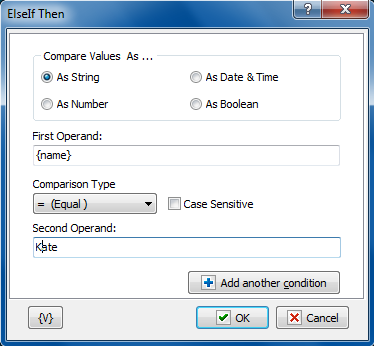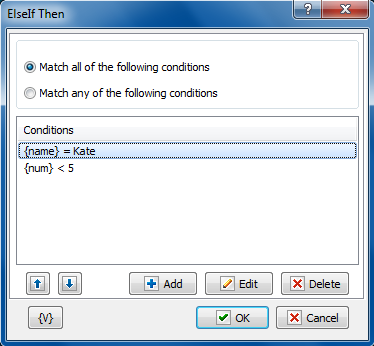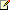﻿ ElseIf Then - RoboTask User's Guide
Keyword Index

 ElseIf Then

This action allows to build a construction of a complex selection with several conditions. For example:

If <condition1> then

DoSomething1

ElseIf <condition2> then

DoSomething2

ElseIf <condition3> then

DoSomething3

.........

Else

DoSomethingN

End if

This action can be put in the block "If Then - EndIf" (between If..Then and End If).

Such construction works in the following way:

 • RoboTask checks the condition . If the condition is fulfilled (the logical value true) then RoboTask performs the steps DoSomething1.
 • Otherwise, RoboTask checks the next condition . If the condition is fulfilled (the logical value True) then RoboTask performs the steps DoSomething2
 • Otherwise, RoboTask checks the next condition . And so on.
 • If none of enumerated conditions is fulfilled, the performing of the task moves to the block Else and then RoboTask performs the steps DoSomethingN.

Of course, it is possible to realize this algorithm by means of actions If..Then, Else, and End If. But apparently such construction is less convenient and less demonstrable. An algorithm with three conditions will look like this:

If <condition1> then

DoSomething1

Else

If <condition2> then

DoSomething2

Else

If <condition3> then

DoSomething3

Else

DoSomethingN

End If

End If

End if

The parameter editing dialog of the action ElseIf Then is completely identical to the window of configuration for the action If Then.Compare Values As

Select the type of data for the expressions that you want to compare.

 • As String - compares two values with alphanumeric characters.
 • As Number - compares two integer values.
 • As Date and Time - compares two date/time values.
 • As Boolean - checks for a true/false or yes/no condition. If you select this option, the If Value Is option appears, allowing you to select the condition of the Boolean.

If Value Is

Appears if you select As Boolean in the Compare Values As area. This option allows you to set the value of the Boolean check. You can select false for certain actions to occur if a condition does not exist. You can select true for certain actions to occur if a condition does exist.

First Operand

Enter the value of the first expression you want to compare.

Comparison Type

Select the operator for the comparison from the following:

 • = Equal to
 • <> Not equal
 • >= More than or equal to
 • > More
 • <= Less than or equal to
 • < Less than

Case Sensitive

Click to make the comparison dependent on whether upper case or lower case characters are used in the expressions.

Second Operand

Enter the value of the second expression you want to compare.

It is possible to use a combination from several conditions in the actions If..Then, ElseIf..Then and While Loop.  To add a condition, press the button Add Another Condition. You will see the similar dialog of editing of the comparison condition.

If you use more than one condition, you will see the list of conditions instead of parameters of conditions.The combination of conditions

Two variants of the combination of conditions are possible.

 • Match all of the following conditions – the common result of the comparing will be True if all enumerated conditions return the result True (logical AND).
 • Match any of the following conditions - the common result of the comparing will be True if at least one of the enumerated conditions returns the result True (logical OR).

Variable

Allows you to add a variable to the action or triggering event that you selected. You must place the cursor in a edit box in the settings dialog window of the action or event and then click the {V} button.Related Topics

File Loop

Text Loop

Dataset Loop

Simple Loop

While loop

Process Loop

End Loop

Break

Continue

If Then

Else

End If

Exit